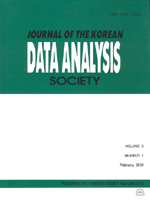상세검색
최근 검색어 전체 삭제
다국어입력
즐겨찾기0KCI등재 학술저널

# Comparisons of Overfitting Probabilities of some Model Selection Criteria in Small Samples

• 등재여부 : KCI등재
• 2006.08
• 1369 - 1380 (12 pages)

We calculated probabilities of overfitting of some model selection criteria, which are AIC, AICc, AICu, SIC, HQ, HQc, Cp, FPE, and FPEu when the sample sizes are 25, 35, 50, and 100. And then, compared these probabilities to find some patterns of overfitting probabilities in some model selection criteria. Overfitting refers to comparing one overfitted model of order k_* + L to the reduced model of order k_*. And suppose there is a true model order k_* and we fit a candidate model of order k_* + L where L>0

1. Introduction

2. Equations of model selection criteria

3. Probabilities of Overfitting

4. Conclusions and Further Research

References